# Maharashtra Board Class 7 Maths Solutions Chapter 8 Algebraic Expressions and Operations on them Practice Set 36

## Maharashtra State Board Class 7 Maths Solutions Chapter 8 Algebraic Expressions and Operations on them Practice Set 36

Question 1.
Simplify (3x – 11y) – (17x + 13y) and choose the right answer.
(A) 7x – 12y
(B) -14x – 54y
(C) -3(5x + 4y)
(D) -2(7x + 12y)
Solution:
(D) -2(7x + 12y)

Hints:
(3x – 11y) – (17x + 13y) = 3x – 11y – 17x – 13y
= – 14x – 24y
= – 2 × 7x – 2 × 12y
= – 2(7x + 12y)

Question 2.
The product of (23x²y³z) and (-15x³yz²) is __
(A) -34x5y4z3
(B) 34x2y3z5
(C) 145x3y2z
(D) 170x3y2z3
Solution:
(A) -34x5y4z3

Question 3.
Solve the following equations:
i. $$4 x+\frac{1}{2}=\frac{9}{2}$$
ii. 10 = 2y + 5
iii. 5m – 4 = 1
iv. 6x – 1 = 3x + 8
v. 2(x – 4) = 4x + 2
vi. 5(x + 1) = 74
Solution:
i. $$4 x+\frac{1}{2}=\frac{9}{2}$$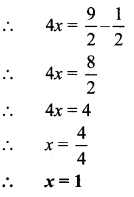ii. 10 = 2y + 5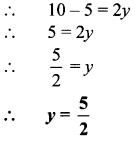iii. 5m – 4 = 1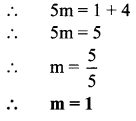iv. 6x – 1 = 3x + 8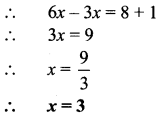v. 2(x – 4) = 4x + 2vi. 5(x + 1) = 74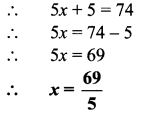Question 4.
Rakesh’s age is less than Sania’s age by 5 years. The sum of their ages is 27 years. How old are they?
Solution:
Let the age of Rakesh be x years.
∴ Sania’s age = (x + 5) years.
According to the given condition,
x + (x + 5) = 27
∴ 2x + 5 = 27
∴ 2x = 27 – 5
∴ 2x = 22
∴ $$x=\frac { 22 }{ 2 }=11$$
Sania’s age = x + 5 = 11 + 5 = 16 years
∴ The ages of Rakesh and Sania are 11 years and 16 years respectively.

Question 5.
When planting a forest, the number of jambhul trees planted was greater than the number of ashoka trees by 60. If there are altogether 200 trees of these two types, how many jambhul trees were planted?
Solution:
Let the number of jambhul trees planted be x.
∴ Number of ashoka trees = x – 60
According to the given condition, x + x – 60 = 200
∴ 2x = 200 + 60
∴ 2x = 260
∴ $$x=\frac { 260 }{ 2 }=130$$
∴ 130 jambhul trees were planted.

Question 6.
Shubhangi has twice as many 20-rupee notes as she has 50-rupee notes. Altogether, she has 2700 rupees. How many 50-rupee notes does she have?
Solution:
Let the number of 50-rupee notes with shubhangi be x.
∴ Number of 20-rupee notes = 2x
∴ Total amount with Shubhangi = Number of 50-rupee notes × 50 + Number of 20-rupee notes × 20
= x × 50 + 2x × 20
= 50x + 40x
= 90x
According to the given condition,
90x = 2700
∴ $$x=\frac { 2700 }{ 90 }=30$$
∴ Shubhangi has 30 notes of 50 rupees.

Question 7.
virat made twice as many runs as Rohit. The total of their scores is 2 less than a double century. How many runs did each of them make?
Solution:
Let the runs made by Rohit be x.
∴ Runs made by Virat = 2x
According to the given condition,
x + 2x = 200 – 2
∴ 3x = 198
∴ $$x=\frac { 198 }{ 3 }=66$$
∴ Runs made by Virat = 2x = 2 × 66 = 132
∴ The runs made by Virat and Rohit are 132 and 66 respectively.

Maharashtra Board Class 7 Maths Chapter 8 Algebraic Expressions and Operations on them Practice Set 36 Intext Questions and Activities

Question 1.
Solve the following equations. (Textbook pg. no. 59)
i. x + 7 = 4
ii. 4p = 12
iii. m – 5 = 4
iv. $$\frac { t }{ 3 }=6$$
Solution:
i. x + 7 = 4
∴ x + 7 – 7 = 4 – 7 ….(Subtracting 7 from both sides)
∴ x + 0 = -3
∴ x = -3

ii. 4p = 12
∴ $$\frac{4 p}{4}=\frac{12}{4}$$ ….(Dividing both sides by 4)
∴ p = 3

iii. m – 5 = 4
∴ m – 5 + 5 = 4 + 5
…. (Adding 5 to both sides)
∴ m + 0 = 9
∴ m = 9

iv. $$\frac { t }{ 3 }=6$$
∴ $$\frac { t }{ 3 }$$ × 3 = 6 × 3 …. (Multiplying both sides by 3)
∴ t = 18